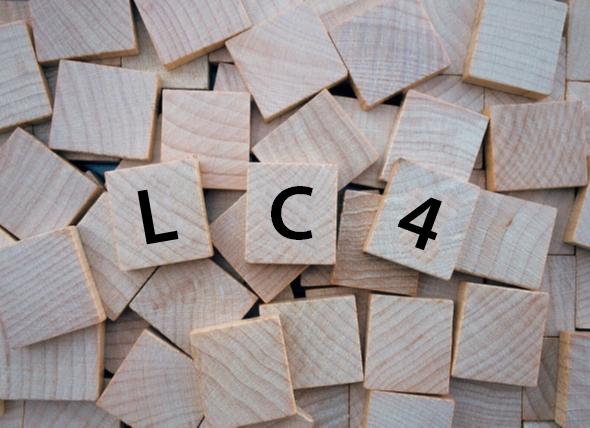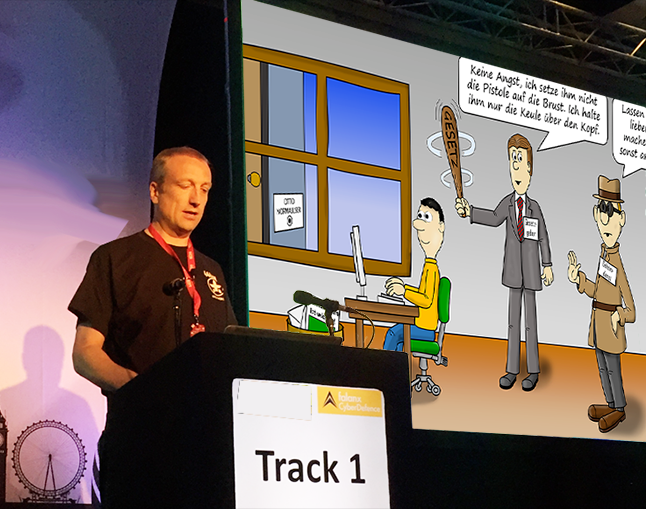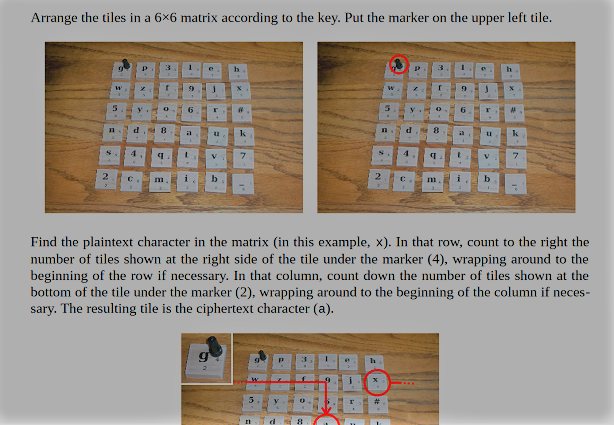# The low-tech cipher LC4LC4 is a cipher that can be computed by hand. According to its creator, it is both secure and easy to use. Is LC4 better than other low-tech ciphers I have introduced on this blog?

Designing a purely manual cipher (i.e., one that can be computed by hand) has proven a difficult problem. Most designs are either too complicated for practical use or insecure (some are even both). Almost all manual ciphers that were developed in the pre-computer era can be broken today with a computer. Although manual encryption algorithms have lost importance with the advent of cheap computers, they are still an active field of research.

### A few good manual ciphers

Here are the best manual cipher designs I am aware of:

• The Double Column Transposition (DCT, also known as “double cube”) is considered a good manual cipher. For the DCT to be secure, both keywords need to have at least 25 letters.
• ADFGVX (a World War I cipher) is another potentially secure manual cipher, provided that the second step of this method (a transposition) is carried out twice.
• There’s an encryption algorithm based on Rubik’s Cube. While this method is not purely manual, it doesn’t require a computer.
• Solitaire is a cipher invented by Bruce Schneier. It uses a deck of cards. While Solitaire is considered secure, it is too complicated for longer messages.
• Handycipher was invented by Bruce Kallick. I blogged about it in 2017.
• Pocket-RC4, Mirdek, and Card-Chameleon are other manual ciphers. I have not looked at them in detail yet.

### LC4

LC4 (also known as “ElsieFour”), developed by Alan Kaminsky, is another cipher that can be computed by hand. LC4 is intended for encrypted communication between humans, and therefore it encrypts plaintexts consisting only of the English letters A through Z plus a few other characters.

LC4 is based on the well-known cipher RC4, which is described in my book Kryptografie – Verfahren, Protokolle, Infrastrukturen. RC4 is probably the simplest modern encryption algorithm that is in wide-spread use. Some programmers can implement it from memory. However, RC4 is still not simple enough to be computed by hand. So, RC is not a manual cipher, but it makes sense to use it as a starting point for designing one. This is what Bruce Kaminsky did.

——————————————–

Next Saturday I will speak at the …History of Cryptography and Codes

Saturday 19 May 2018, Birkbeck, London

——————————————–

### How LC4 works

LC4 encrypts plaintexts consisting of the following 36 characters:

# _ 2 3 4 5 6 7 8 9 a b c d e f g h i j k l m n o p q r s t u v w x y z

Internally, these characters are treated as numbers 0 through 35. The character # is used, rather than the digit 0, to avoid confusion with the uppercase letter O. The character _ is used, rather than the digit 1, to avoid confusion with the uppercase letter I and the lowercase letter l. The # and _ characters can be used as separators in the plaintext.

The key LC4 uses is a permutation of the integers 0 through 35 (or of the 36 characters mentioned above). Here is an example key:

`hwqnazb3x_ie497g5oysltcvfprd82u#6jmk`

The size of the key space is 36!, which is equivalent to a 138-bit key.

LC4 uses a 6×6 matrix S (“state matrix”) containing a permutation of the integers 0 through 35, as well as two indexes i and j. These indexes are used to define a matrix element S[i][j] (the first index is the row, the second index is the column).

Before the actual encryption starts, the state matrix is initialized as follows:

`For k=0 to 35: S[k/6][k mod 6] = K[k]`

To encrypt, for each plaintext character P the following steps are performed:

```r = row of S in which P appears (0 ≤ r ≤ 5)
c = column of S in which P appears (0 ≤ c ≤ 5)
x = (r + (S[i][j] / 6)) mod 6
y = (c + (S[i][j] mod 6)) mod 6
C = S[x][y]
Output C
Right-rotate row r of S
Down-rotate column y of S
i = (i + (C / 6)) mod 6
j = (j + (C mod 6)) mod 6```

C is the ciphertext character.

The “Right-rotate row r” subroutine is:

```(S[r], S[r], S[r], S[r], S[r], S[r]) = (S[r], S[r], S[r], S[r], S[r], S[r])
c ← (c + 1) mod 6
If x = r: y = (y + 1) mod 6
If i = r: j = (j + 1) mod 6```

The “Down-rotate column y” subroutine is:

```(S[y], S[y], S[y], S[y], S[y], S[y]) = (S[y], S[y], S[y], S[y], S[y], S[y])x ← (x + 1) mod 6
If c = y: r = (r + 1) mod 6
If j = y: i = (i + 1) mod 6```

This algorithm may look complicated, but in his paper (chaper 6) Kaminski explaines how these steps can be easliy carried out by hand.### An LC4 challenge

LC4 is one of the many encryption algotithms implemented in the open source software CrypTool 2.

There are a number of LC4 challenges available on the crypto puzzle portal MysteryTwister C3. Three of them refer to weakened LC4 variants. A fourth MTC3 challenge is about solving a two-part cryptogram enciphered with an unweakened LC4. Here is the first ciphertext part:

`6r4g6kfg5_3dyru5##bypmcsfpfs9f6m4c5apxzflnogloqwnoe`

And here’s the second part:

```bcad6safmyscvsj93p3932kxsn556bzjinmzmhmouca#2oa7teq
63d5ntvb347eo965hj7y4kkk8kxp#vi_rvuki9d87rjdxfort5x
hmaqqmvn#_amcwrckduqzrxy_w65k3avifquzjjaovoeosrswt6
_gf6d8dv6_x3jpmdio_76rknonnxf```

Before you try to solve this challenge you should take a look the details here. This LC4 challenge is MTC3 level 3, which means that it is difficult. So far, no MTC3 participant has solved it. Perhaps, a reader of this blog will be the first.

Further reading: The Top 50 unsolved encrypted messages: 48. Ron Rivest’s timelock cryptogram

## Subscribe to Blog via Email

1. #1 George Lasry